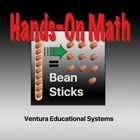Hands-On Math: Bean Sticks

• SEARCH TYPE

All iPhone applications categories

All iPhone games categories# Hands-On Math: Bean Sticks

0 4

4 Users
rating

## Screenshots

Description

Hands-On Math: Bean Sticks is an interactive learning environment where students explore and discover place value, and addition and subtraction with regrouping. Bean Sticks are used to model the decimal place value system. Math manipulatives such as Bean Sticks allow students to experience and develop math concepts through interaction and discovery. Concept development is the foundation of mathematical understanding.

Hands-On Math: Bean Sticks simulates the use of a popular math manipulative used to teach place value and addition and subtraction with regrouping.

Bean Sticks are one of the best ways to help students place value. Using this app students place Bean Sticks on an interactive Playground to represent numbers. Bean Sticks can be regrouped or exchanged by dragging a virtual manipulative from one column to the next.

Using Hands-On Math: Bean Sticks students can develop the following important mathematical concepts:

* Place value concepts
* Regrouping in the ones, tens and hundreds places
* Basic operations: addition and subtraction
* Naming numbers
* Expanded notation